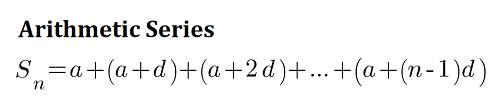# Sum of Series Calculator

Reviewed by Wojciech Sas, PhD candidate
Last updated: Jun 13, 2022

Using the sum of series calculator, you can calculate the sum of an infinite series that has a geometric convergence as well as the partial sum of an arithmetic or geometric series. This summation solver can also help you calculate the convergence or divergence of a series.

## How do I calculate the sum of a series?

Many a time, we'd want to calculate the sum of a series, and, in order to do that, it helps to first know if the series is arithmetic or geometric. In an arithmetic series, the difference between each pair of successive terms is constant, while in a geometric series the ratio between each pair of successive terms is constant.

For instance, let's consider the following series of the first 10 odd numbers:
1 + 3 + 5 + 7 + 9 + 11 + 13 + 15 + 17 + 19

This is an arithmetic series since the difference between any two successive pairs of numbers is 2. We can find the sum by using the following formula:

$S_n = \frac{n}{2}\ [2a + (n - 1) d]$,

where:

n - Number of terms;
a - First term; and
d - Common difference.

We can use the above formula also to calculate the partial sum of an infinite arithmetic series. So in the above example, the sum to 10 terms will be:

$S_{10} = \frac{10}{2}\ [2\times1 + (10 - 1)\times2]$

$S_{10} = 100$

If we have a geometric series, we'd use a different formula to find the sum, which we'll take a look at below.

## How do I calculate the sum of a geometric series?

To know how to find the sum of a series in geometric progression, we can use either the finite sum formula or the infinite sum calculation. A geometric series can converge or diverge depending on the value of the common ratio r.

To decide on the convergence vs. divergence of a geometric series, we'd follow the following guideline based on the common ratio r:

• If |r| > 1, then the geometric series diverges and its sum to infinite terms can't be determined;
• If |r| < 1, then the geometric series converges to a finite sum and we can calculate the sum of the infinite series; and
• If |r| = 1, then the geometric series is periodic and its sum to infinite terms can't be determined.

On the other hand, to calculate the partial sum of a geometric series to a specific number of terms, we will use the formula:

$S_n = \frac{a(1 - r^n)}{1 - r}$,

where

a - First term;
r - Common ratio; and
n - Number of terms.

## How do I calculate the sum of an infinite geometric series?

To calculate the sum of a series with a geometric convergence to an infinite number of terms, we will use the formula:

$S = \frac{a}{1 - r}$

where:

a - First term; and
r - Common ratio.

For example, consider the following geometric series:

$1 + \frac{1}{2} + \frac{1}{4} + \frac{1}{8} + ...$

Here, a = 1 and r = $\frac{1}{2}$.

So, the sum to an infinite number of terms is:

$S = \frac{1}{1 - \frac{1}{2}}$,

which gives us

S = 2.

In this manner, we can calculate the sum of a geometric series with an infinite number of terms if the common ratio r is between -1 and 1.

## FAQ

### How do I calculate the convergence or divergence of a series?

To decide on the convergence vs. divergence of an infinite geometric series, we follow these steps:

1. Determine the common ratio r.
2. If |r| > 1, then the series diverges.
3. If |r| < 1, then the series converges.
4. If |r| = 1, then the series is periodic, but its sum diverges.

### What is the formula for the sum of n terms of an arithmetic progression?

Sn = (n/2)×[2a + (n-1)×d] is the formula to find the sum of n terms of an arithmetic progression, where:

• n is the number of terms;
• a is the first term; and
• d is the common difference, or the difference between successive terms.

### What is the sum of series formula for an arithmetic progression?

Sn = (n/2)×(a + l), which means we can find the sum of an arithmetic series by multiplying the number of terms by the average of the first and last terms.

• n is the number of terms;
• a is the first term; and
• l is the last term.

### What is the sum of 1 to N?

1 + 2 + 3 + ... + N = N(N + 1) / 2

We can use this formula to find the sum of the first N natural numbers. This formula results from the sum of the arithmetic progression formula, with the first term as 1 and the common difference as 1.

Series type
ArithmeticFirst term (a)
Common difference (d)
Number of terms (n)
Arithmetic series sum (Sₙ)
People also viewed…

### Area of a rectangle

Area of a rectangle calculator finds the area, perimeter and diagonal of a rectangle.

### Grams to cups

The grams to cups calculator converts between cups and grams. You can choose between 20 different popular kitchen ingredients or directly type in the product density.

### Podcasts

Do you feel like you could be doing something more productive or educational while on a bus? Or while cleaning the house? Well, why don't you dive into the rich world of podcasts! With this podcast calculator, we'll work out just how many great interviews or fascinating stories you can go through by reclaiming your 'dead time'!

### Solving by completing the square

We explain how to solve a quadratic equation by completing the square.